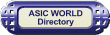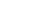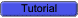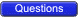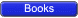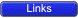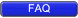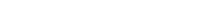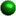Binary Codes

Binary codes are codes which are represented in binary system with modification from the original ones. Below we will be seeing the following:• Weighted Binary Systems
• Non Weighted CodesWeighted Binary Systems

Weighted binary codes are those which obey the positional weighting principles, each position of the number represents a specific weight. The binary counting sequence is an example.Decimal 8421 2421 5211 Excess-3 0 0000 0000 0000 0011 1 0001 0001 0001 0100 2 0010 0010 0011 0101 3 0011 0011 0101 0110 4 0100 0100 0111 0111 5 0101 1011 1000 1000 6 0110 1100 1010 1001 7 0111 1101 1100 1010 8 1000 1110 1110 1011 9 1001 1111 1111 11008421 Code/BCD Code

The BCD (Binary Coded Decimal) is a straight assignment of the binary equivalent. It is possible to assign weights to the binary bits according to their positions. The weights in the BCD code are 8,4,2,1.Example: The bit assignment 1001, can be seen by its weights to represent the decimal 9 because:1x8+0x4+0x2+1x1 = 92421 Code

This is a weighted code, its weights are 2, 4, 2 and 1. A decimal number is represented in 4-bit form and the total four bits weight is 2 + 4 + 2 + 1 = 9. Hence the 2421 code represents the decimal numbers from 0 to 9.5211 Code

This is a weighted code, its weights are 5, 2, 1 and 1. A decimal number is represented in 4-bit form and the total four bits weight is 5 + 2 + 1 + 1 = 9. Hence the 5211 code represents the decimal numbers from 0 to 9.Reflective Code

A code is said to be reflective when code for 9 is complement for the code for 0, and so is for 8 and 1 codes, 7 and 2, 6 and 3, 5 and 4. Codes 2421, 5211, and excess-3 are reflective, whereas the 8421 code is not.Sequential Codes

A code is said to be sequential when two subsequent codes, seen as numbers in binary representation, differ by one. This greatly aids mathematical manipulation of data. The 8421 and Excess-3 codes are sequential, whereas the 2421 and 5211 codes are not.Non Weighted Codes

Non weighted codes are codes that are not positionally weighted. That is, each position within the binary number is not assigned a fixed value.Excess-3 Code

Excess-3 is a non weighted code used to express decimal numbers. The code derives its name from the fact that each binary code is the corresponding 8421 code plus 0011(3).Example: 1000 of 8421 = 1011 in Excess-3Gray Code

The gray code belongs to a class of codes called minimum change codes, in which only one bit in the code changes when moving from one code to the next. The Gray code is non-weighted code, as the position of bit does not contain any weight. The gray code is a reflective digital code which has the special property that any two subsequent numbers codes differ by only one bit. This is also called a unit-distance code. In digital Gray code has got a special place.Decimal Number Binary Code Gray Code 0 0000 0000 1 0001 0001 2 0010 0011 3 0011 0010 4 0100 0110 5 0101 0111 6 0110 0101 7 0111 0100 8 1000 1100 9 1001 1101 10 1010 1111 11 1011 1110 12 1100 1010 13 1101 1011 14 1110 1001 15 1111 1000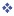Binary to Gray Conversion• Gray Code MSB is binary code MSB.
• Gray Code MSB-1 is the XOR of binary code MSB and MSB-1.
• MSB-2 bit of gray code is XOR of MSB-1 and MSB-2 bit of binary code.
• MSB-N bit of gray code is XOR of MSB-N-1 and MSB-N bit of binary code.